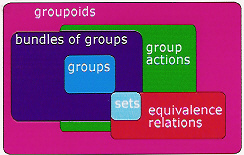# In a groupoid, do any two objects have a morphism between them?

This is a question about the definition of a groupoid (in category theory).

I've read the Wikipedia article, and it says that a groupoid is a small category in which every morphism is an isomorphism. That doesn't imply that each two objects have a morphism between them. For example, I can take a category with two objects and two morphisms. This will necessarily be a small category with every morphism being an isomorphism, but there can't be any morphisms between the two objects.

However, the article also says that groupoids were intruduced by Heinrich Brandt, via Brandt semigroups. This seems to be false because what Brandt introduced wasn't closed under multiplication, according to Clifford and Preston's The Algebraic Theory of Semigroups, and it was the authors who thought closing it would be a good idea. But this is not my problem.

They give Brandt's axioms:

Let $B$ be a set with a partial operation written multiplicatively. Then $B$ is a groupoid when:

$(B1)$ if $ab=c$, then each of the three elements is uniquely determined by the other two;

$(B2)$

• if $ab$ and $bc$ are defined, so are $(ab)c$ and $a(bc),$ and these are equal;

• if $ab$ and $(ab)c$ are defined, so are $bc$ and $a(bc),$ and $(ab)c=a(bc);$

• if $bc$ and $a(bc)$ are defined, so are $ab$ and $(ab)c,$ and $(ab)c=a(bc);$

$(B3)$ to each $a$ there correspond unique $e,f$ and $a'$ such that $ea=af=a$ and $aa'=f;$

$(B4)$ if $e^2=e$ and $f^2=f,$ there exists $a$ such that $ea=af=a.$

I'm inclined to believe that the first three axioms are equivalent to what Wikipedia says. I haven't checked very carefully, but it looks all right. But the fourth axiom, as I understand it, says that any two identity morphisms must be "connected" by some third morphism, which means that there's a morphism between any two objects.

It doesn't seem very much to demand of a groupoid to satisfy this axiom I think. The only groupoids I know are the fundamental groupoids in topology. It satisfies the axiom when the space is path-connected, so not too much.

But which is the right definition?

• Did you mean groupoid in your entire post? – Amzoti Apr 5 '13 at 4:05
• @Amzoti Yes, sorry, non-native mistake. I'll edit that. – Bartek Apr 5 '13 at 4:07
• no problem, I make dozens of typos daily! :) Regards – Amzoti Apr 5 '13 at 4:09

## 2 Answers

The first definition is correct. The second is too restrictive.

The disadvantages of the restriction to "connected", also called "transitive", groupoids was early recognised. The wider definition allows for the following diagram:For more information, see my groupoids web page.